Question

# A 600 VA, 60 Hz, 220/12 Vrms transformer is connected as an autotransformer. Assuming the transformer...

A 600 VA, 60 Hz, 220/12 Vrms transformer is connected as an autotransformer. Assuming the transformer is ideal, determine a) the output voltage, b) the maximum V-A load rating possible, c) and the maximum input current, d) Determine the input current if a 50 Ohm resistor is connected on the output with 220 V applied to the primary.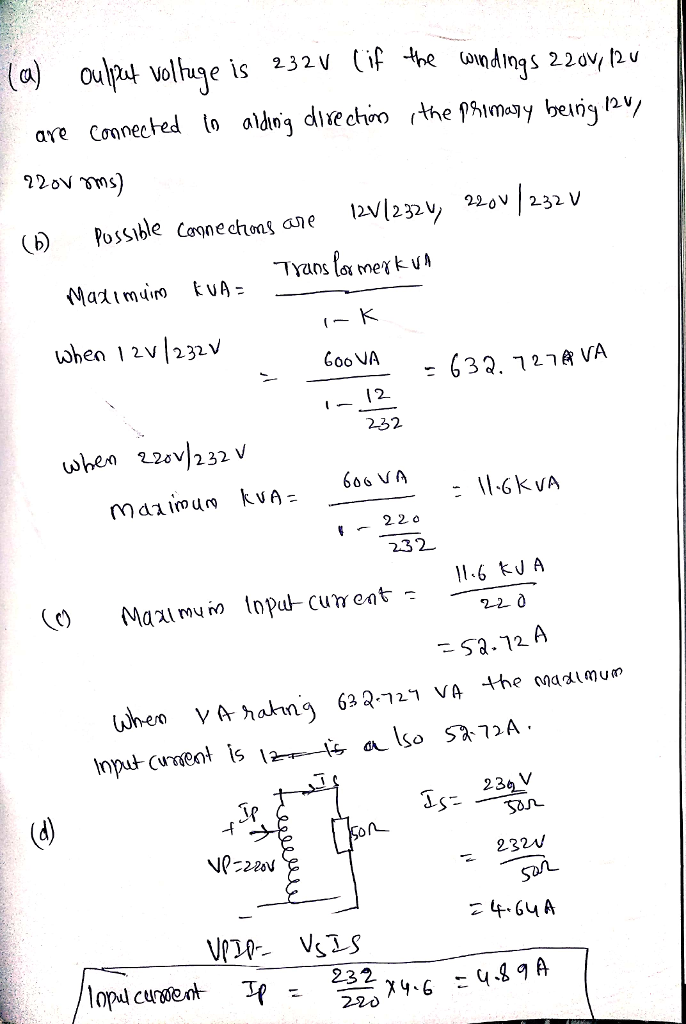#### Earn Coins

Coins can be redeemed for fabulous gifts.

Similar Homework Help Questions
• ### Ons Nse-12) Nc(-120) Q1: A 100 VA 120/12-V transformer is to be connected so as to form a step-up...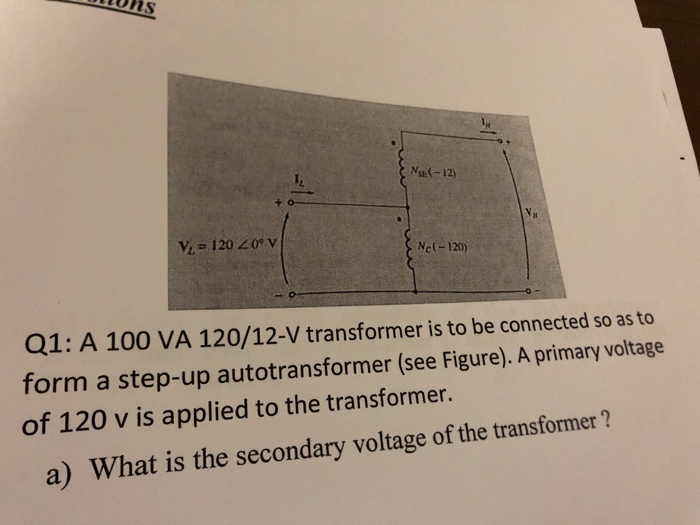ons Nse-12) Nc(-120) Q1: A 100 VA 120/12-V transformer is to be connected so as to form a step-up autotransformer (see Figure). A primary voltage of 120 v is applied to the transformer. a) What is the secondary voltage of the transformer? Q1: A 100 VA 120/12-V transformer is to be connected so as to form a step-up autotransformer (see Figure). A primary voltage of 120 v is applied to the transformer. a) What is the secondary voltage of the...

• ### A single phase 60 Hz two-winding power transformer is rated at 2400V/240V and 100 kVA with...

A single phase 60 Hz two-winding power transformer is rated at 2400V/240V and 100 kVA with Zeq = 0.5 + j0.8 Ohm. If an autotransformer is built from this conventional transformer to supply a load at 2640V with supply voltage 2400V. Draw the autotransformer and calculate Zeq, the voltage, current and apparent power rating of the auto transformer. Also calculate the apparent power rating advantage of autotransformer over conventional transformer.

• ### An autotransformer with a total of 600 turns has its secondary embracing 200 turns. The 600...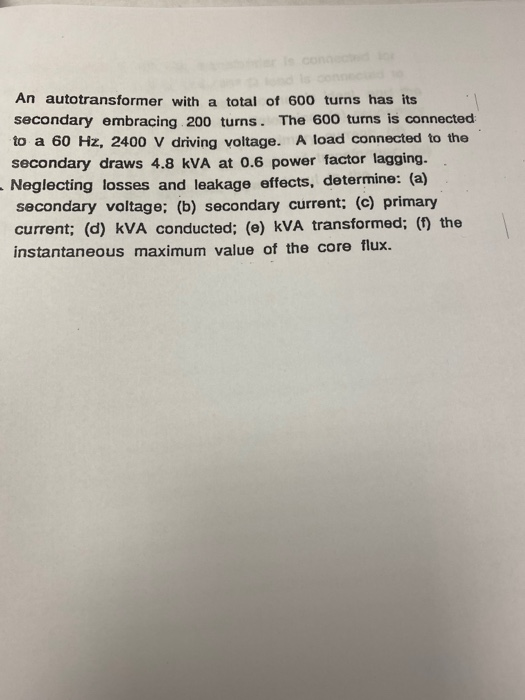An autotransformer with a total of 600 turns has its secondary embracing 200 turns. The 600 turns is connected to a 60 Hz, 2400 V driving voltage. A load connected to the Secondary draws 4.8 kVA at 0.6 power factor lagging. Neglecting losses and leakage effects, determine: (a) secondary voltage; (b) secondary current; (c) primary current; (d) KVA conducted; (e) KVA transformed; (0) the instantaneous maximum value of the core flux.

• ### Question 1 220 Vrms 50 Hz 10:1 CR Figure 1 Figure 1 shows the circuit diagram...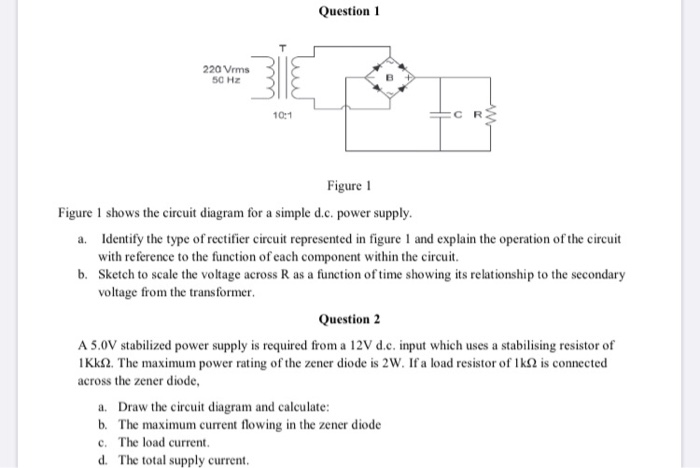Question 1 220 Vrms 50 Hz 10:1 CR Figure 1 Figure 1 shows the circuit diagram for a simple d.c. power supply. a. Identify the type of rectifier circuit represented in figure I and explain the operation of the circuit with reference to the function of each component within the circuit. b. Sketch to scale the voltage across R as a function of time showing its relationship to the secondary voltage from the transformer. Question 2 A 5.0V stabilized power...

• ### A single-phase 10 kVA, 460/120 V, 60 Hz, transformer has an efficiency of 96% when it...

A single-phase 10 kVA, 460/120 V, 60 Hz, transformer has an efficiency of 96% when it delivers 9 kW at 0.9 pf lagging. The transformer is connected as an autotransformer to supply load to a 460 V circuit from a 580 V source. Show the autotransformer connection Determine kVA rating of the autotransformer Determine the efficiency of the autotransformer at full-load with 0.9 pf lagging

• ### Q-1 A 60Hz, 480V/240V,960V A transformer is supplying a 960W passive load at 240V. The resistances...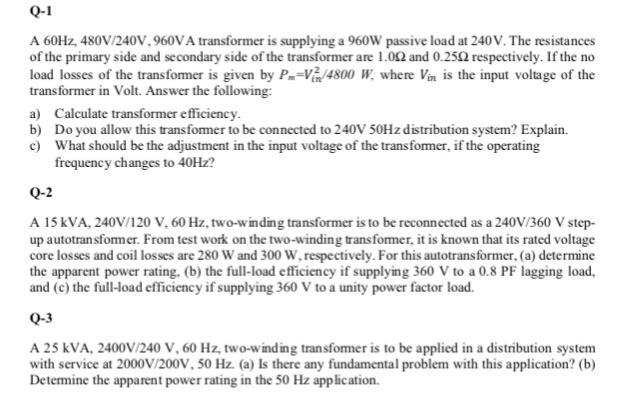Q-1 A 60Hz, 480V/240V,960V A transformer is supplying a 960W passive load at 240V. The resistances of the primary side and secondary side of the transformer are 1.092 and 0.252 respectively. If the no load losses of the transfomer is given by P-Vã 4800 W where Vn is the input voltage of the transformer in Volt. Answer the following: a) Calculate transformer efficiency b) Do you allow this transformer to be connected to 240V 50Hz distribution system? Explain. c What...

• ### A single phase 60 Hz two-winding power transformer is rated at 2400V/480V and 150 kVA. If...

A single phase 60 Hz two-winding power transformer is rated at 2400V/480V and 150 kVA. If an autotransformer is built from this conventional transformer to supply a load at 2880V with supply voltage 2400V. Draw the autotransformer and calculate the voltage, current and apparent power rating of the auto transformer. Also calculate the apparent power rating advantage of autotransformer over conventional transformer.

• ### Problem 5: A single phase 60 Hz two-winding power transformer is rated at 2400V/480V and 150...

Problem 5: A single phase 60 Hz two-winding power transformer is rated at 2400V/480V and 150 kVA. If an autotransformer is built from this conventional transformer to supply a load at 2880V with supply voltage 2400V. Draw the autotransformer and calculate the voltage, current and apparent power rating of the auto transformer. Also calculate the apparent power rating advantage of autotransformer over conventional transformer.

• ### A single-phase transformer of nominal values of 10kVA, 440/220 V and 60 Hz supplies nominal load...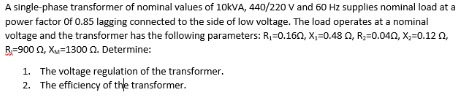A single-phase transformer of nominal values of 10kVA, 440/220 V and 60 Hz supplies nominal load at a power factor of 0.85 lagging connected to the side of low voltage. The load operates at a nominal voltage and the transformer has the following parameters: R=0.160, X,=0.48 , R=0.040, X=0.12, R900, Xy=1300. Determine: 1. The voltage regulation of the transformer. 2. The efficiency of the transformer.

• ### Polar form please For problems 1-5 below, use the autotransformer diagram below. The transformer is wired...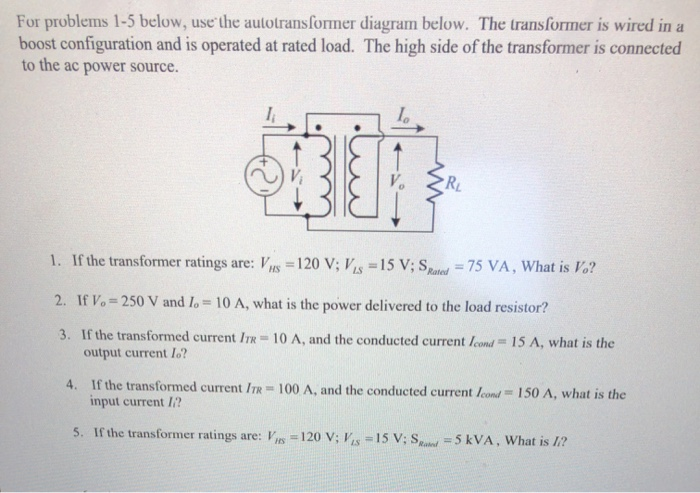Polar form please For problems 1-5 below, use the autotransformer diagram below. The transformer is wired in a boost configuration and is operated at rated load. The high side of the transformer is connected to the ac power source. 1. If the transformer ratings VIS = 120 V; VS =15 V; S are: 75 VA, What is Vo? Rated 2. If Vo 250 V and lo 10 A, what is the power delivered to the load resistor? 3. If the...